# Equation with fractions

What is another way to write the equation 7/8 x + three-fourths = negative 6?

x =  -7.7143

### Step-by-step explanation: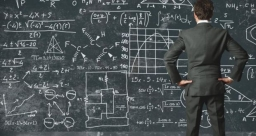Did you find an error or inaccuracy? Feel free to write us. Thank you!Tips to related online calculators
Need help to calculate sum, simplify or multiply fractions? Try our fraction calculator.
Do you have a linear equation or system of equations and looking for its solution? Or do you have a quadratic equation?

## Related math problems and questions:

• Patel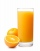Patel squeezed oranges so that his family could have fresh-squeezed juice for breakfast. He squeezed 4/17 cups from the first orange, 3/10 cups from the second orange, StartFraction 9 over 20 E
• The number 4Two-thirds of a number is negative six. Find the number.
• Job applicantsJob applicants: three-fourths of applicants had experience for a position. The number that did not have prior experience was 36. How many people applied for the job?
• Write 2Write 791 thousandths as fraction in expanded form.
• Negative in equation2x + 3 + 7x = – 24, what is the value of x?
• Equation with fractionsSolve an equation: 1- (2x-5)/(6) =3- (x)/(4) It is an equation with fractions.
• BoatsThree-quarters of boats are white, 1/7 are blue and 9 are red. How many boats do we have?
• Z7-I-4 stars 4949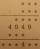Write instead of stars digits so the next write of product of the two numbers to be valid: ∗ ∗ ∗ · ∗ ∗ ∗ ∗ ∗ ∗ ∗ 4 9 4 9 ∗ ∗ ∗ ∗ ∗ ∗ 4 ∗ ∗
• Equivalent fractions 2Write the equivalent multiplication expression. 2 1/6÷3/4
• Two identical five-digitsWe have two identical five-digit numbers, but we don't know which ones. When we write one before the first and after the second one we get two six-digit numbers, one of which is three times the other. What are the numbers?
• Eq-fracSolve the following equation with fractions: h + 1/3 =5/3
• EqnSolve equation with fractions: 2x/3-50=40+x/4
• Product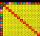The sum and the product of three integers are 6. Write largest of them.
• Even / odd numbers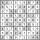a / Using variable n write two consecutive odd numbers. b / the sum of three consecutive odd numbers is 333. What are this numbers?
• Recipe ingredients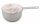Monica’s cookie recipe calls for Three-fourths of a cup of flour. Her mother’s recipe calls for Two-thirds as much as Monica’s. How many cups of flour does her mother’s recipe require?
• Math homeworkIt took Jose two-thirds of an hour to complete his math homework on Monday, three-fourths of an hour on Tuesday, any two- fifths of an hour on Wednesday. How many hours did it take Jose to complete his homework altogether?
• Ratio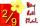Write the ratio with other numbers so that the value is the same: 2: 9